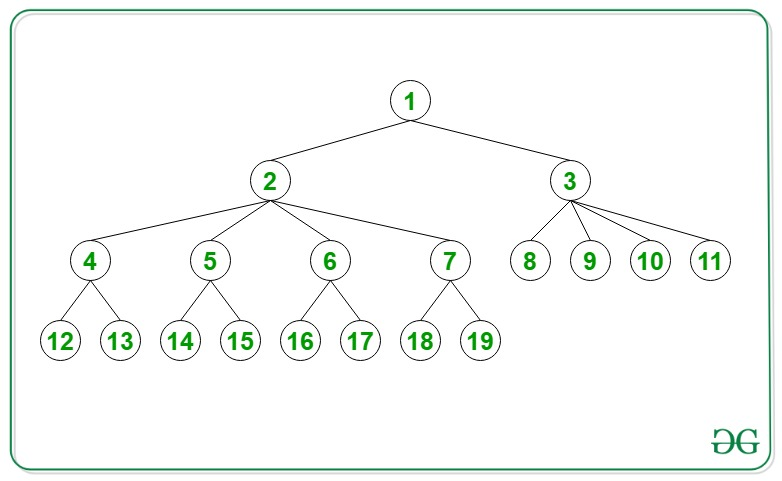# Path from the root node to a given node in an N-ary Tree

• Difficulty Level : Medium
• Last Updated : 19 Jan, 2021

Given an integer N and an N-ary Tree of the following form:

• Every node is numbered sequentially, starting from 1, till the last level, which contains the node N.
• The nodes at every odd level contains 2 children and nodes at every even level contains 4 children.

The task is to print the path from the root node to the node N.

Attention reader! Don’t stop learning now. Get hold of all the important DSA concepts with the DSA Self Paced Course at a student-friendly price and become industry ready.  To complete your preparation from learning a language to DS Algo and many more,  please refer Complete Interview Preparation Course.

In case you wish to attend live classes with experts, please refer DSA Live Classes for Working Professionals and Competitive Programming Live for Students.

Examples:

Input: N = 14Output: 1 2 5 14
Explanation: The path from node 1 to node 14 is 1 – > 2 – > 5 – > 14.

Input: N = 11
Output: 1 3 11
Explanation: The path from node 1 to node 11 is 1 – > 3 – > 11.

Approach: Follow the steps below to solve the problem:

• Initialize an array to store the number of nodes present in each level of the Tree, i.e. {1, 2, 8, 16, 64, 128 ….} and store it.
• Calculate prefix sum of the array i.e. {1 3 11 27 91 219 …….}
• Find the index ind in the prefix sum array which exceeds or is equal to N using lower_bound(). Therefore, ind indicates the number of levels that need to be traversed to reach node N.
• Initialize a variable say, temp = N and an array path[] to store the nodes from root to N.
• Decrement ind until it is less than or equal to 1 and keep updating val = temp – prefix[ind – 1].
• Update temp = prefix[ind – 2] + (val + 1) / 2 if ind is odd.
• Otherwise, update temp = prefix[ind – 2] + (val + 3) / 4 if ind is even.
• Append temp into the path[] array.
• Finally, print the array, path[].

Below is the implementation of the above approach:

## C++

 `// C++ program for the above approach``#include ``using` `namespace` `std;``typedef` `long` `long` `ll;` `// Function to find the path``// from root to N``void` `PrintPathNodes(ll N)``{` `    ``// Stores the number of``    ``// nodes at (i + 1)-th level``    ``vector arr;``    ``arr.push_back(1);` `    ``// Stores the number of nodes``    ``ll k = 1;` `    ``// Stores if the current``    ``// level is even or odd``    ``bool` `flag = ``true``;` `    ``while` `(k < N) {` `        ``// If level is odd``        ``if` `(flag == ``true``) {``            ``k *= 2;``            ``flag = ``false``;``        ``}` `        ``// If level is even``        ``else` `{` `            ``k *= 4;``            ``flag = ``true``;``        ``}` `        ``// If level with``        ``// node N is reached``        ``if` `(k > N) {``            ``break``;``        ``}` `        ``// Push into vector``        ``arr.push_back(k);``    ``}` `    ``ll len = arr.size();``    ``vector prefix(len);``    ``prefix = 1;` `    ``// Compute prefix sums of count``    ``// of nodes in each level``    ``for` `(ll i = 1; i < len; ++i) {``        ``prefix[i] = arr[i] + prefix[i - 1];``    ``}` `    ``vector::iterator it``        ``= lower_bound(prefix.begin(),``                      ``prefix.end(), N);` `    ``// Stores the level in which``    ``// node N s present``    ``ll ind = it - prefix.begin();` `    ``ll temp = N;` `    ``// Store path``    ``vector<``int``> path;` `    ``path.push_back(N);` `    ``while` `(ind > 1) {``        ``ll val = temp - prefix[ind - 1];` `        ``if` `(ind % 2 != 0) {``            ``temp = prefix[ind - 2]``                   ``+ (val + 1) / 2;``        ``}``        ``else` `{``            ``temp = prefix[ind - 2]``                   ``+ (val + 3) / 4;``        ``}``        ``--ind;` `        ``// Insert temp into path``        ``path.push_back(temp);``    ``}` `    ``if` `(N != 1)``        ``path.push_back(1);` `    ``// Print path``    ``for` `(``int` `i = path.size() - 1;``         ``i >= 0; i--) {` `        ``cout << path[i] << ``" "``;``    ``}``}` `// Driver Code``int` `main()``{` `    ``ll N = 14;` `    ``// Function Call``    ``PrintPathNodes(N);` `    ``return` `0;``}`

## Python3

 `# Python3 program for the above approach``from` `bisect ``import` `bisect_left` `# Function to find the path``# from root to N``def` `PrintPathNodes(N):` `    ``# Stores the number of``    ``# nodes at (i + 1)-th level``    ``arr ``=` `[]``    ``arr.append(``1``)` `    ``# Stores the number of nodes``    ``k ``=` `1` `    ``# Stores if the current``    ``# level is even or odd``    ``flag ``=` `True``    ``while` `(k < N):` `        ``# If level is odd``        ``if` `(flag ``=``=` `True``):``            ``k ``*``=` `2``            ``flag ``=` `False` `        ``# If level is even``        ``else``:``            ``k ``*``=` `4``            ``flag ``=` `True` `        ``# If level with``        ``# node N is reached``        ``if` `(k > N):``            ``break` `        ``# Push into vector``        ``arr.append(k)``    ``lenn ``=` `len``(arr)``    ``prefix ``=` `[``0``]``*``(lenn)``    ``prefix[``0``] ``=` `1` `    ``# Compute prefix sums of count``    ``# of nodes in each level``    ``for` `i ``in` `range``(``1``,lenn):``        ``prefix[i] ``=` `arr[i] ``+` `prefix[i ``-` `1``]``    ``it ``=` `bisect_left(prefix, N)` `    ``# Stores the level in which``    ``# node N s present``    ``ind ``=` `it``    ``temp ``=` `N` `    ``# Store path``    ``path ``=` `[]``    ``path.append(N)``    ``while` `(ind > ``1``):``        ``val ``=` `temp ``-` `prefix[ind ``-` `1``]` `        ``if` `(ind ``%` `2` `!``=` `0``):``            ``temp ``=` `prefix[ind ``-` `2``] ``+` `(val ``+` `1``) ``/``/` `2``        ``else``:``            ``temp ``=` `prefix[ind ``-` `2``] ``+` `(val ``+` `3``) ``/``/` `4``        ``ind ``-``=` `1` `        ``# Insert temp into path``        ``path.append(temp)``    ``if` `(N !``=` `1``):``        ``path.append(``1``)` `    ``# Print path``    ``for` `i ``in` `range``(``len``(path)``-``1``, ``-``1``, ``-``1``):``        ``print``(path[i], end``=``" "``)` `# Driver Code``if` `__name__ ``=``=` `'__main__'``:``    ``N ``=` `14` `    ``# Function Call``    ``PrintPathNodes(N)` `    ``# This code is contributed by mohit kumar 29`
Output:
`1 2 5 14`

Time Complexity: O(log(N))
Auxiliary Space: O(log(N))

My Personal Notes arrow_drop_up